• PMVS算法的CPU多线程和GPU两级粒度并行策略.pdf
• 三维重建（四）PMVS算法 the patch-based MVS algorithm 通过前面的介绍，已经获得了相机的参数，我们可以利用这些参数使用基于面片的三维多视角立体视觉算法（PMVS）重建出稠密的点云。下面详细介绍一下PMVS算法。 ...
三维重建（四）PMVS算法 the patch-based MVS algorithm 通过前面的介绍，已经获得了相机的参数，我们可以利用这些参数使用基于面片的三维多视角立体视觉算法（PMVS）重建出稠密的点云。下面详细介绍一下PMVS算法。 一、基本概念介绍 1、面片（patch） 面片p是一个近似的正切与重建物体表面的一个小矩形，他的一边平行于参考相机的x轴。对于一个面片p，他的几何特征如下： 中心点：c§； 单位法向：n§，该向量指向相机的光心； 面片p对应一个参考图像R§，在R§中p是可见的。针对p有扩展矩形，p在R§中的投影是μ×μ大小的，在原论文中μ=5 or 7
2、灰度一致性函数（Photometric Discrepancy Function）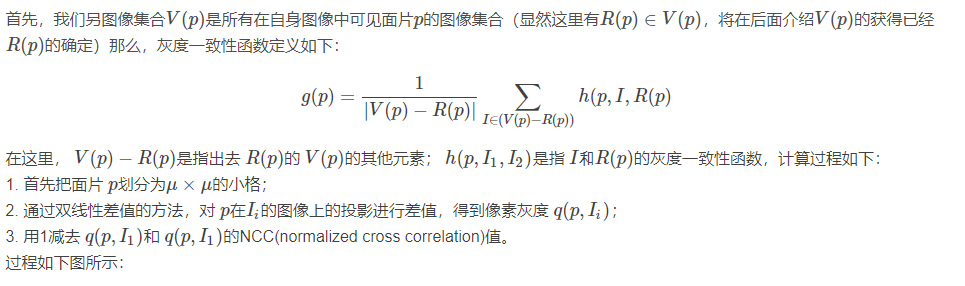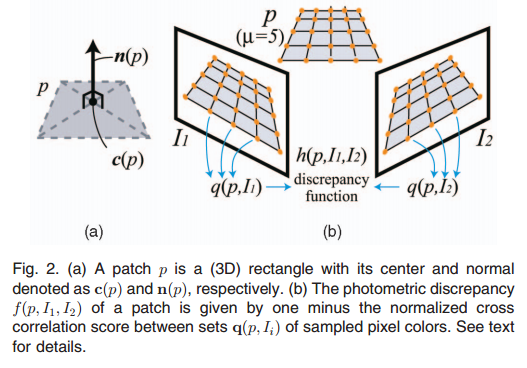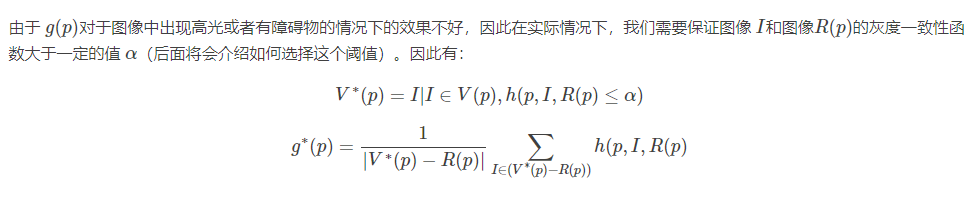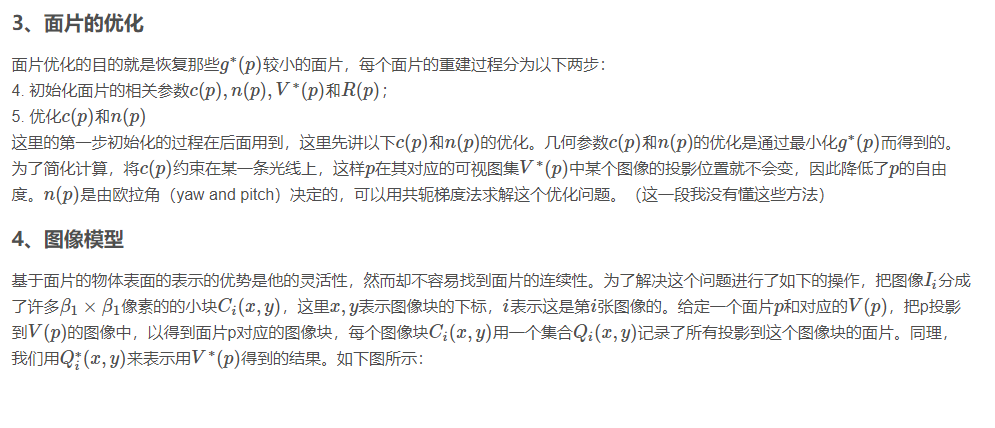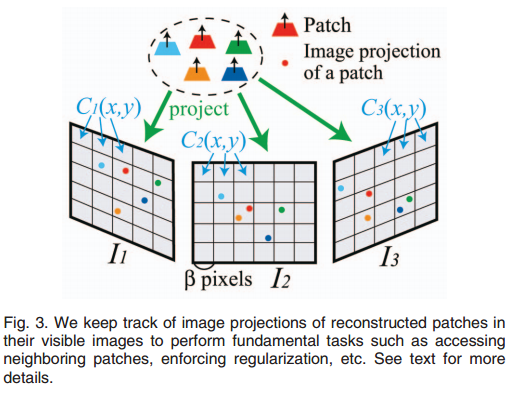二、面片的重构 基于面片的多视角三维立体视觉算法的目的就是保证在每个图像块Ci(x,y)上至少有一个面片的的投影。主要分为以下三步：
初始化特征匹配（initial feature matching）；面片生成（patch expansion）；面片筛选（patch filtering）； 初始化特征匹配的目的就是生成一系列稀疏的面片，面片的生成和筛选都要执行n次使得面片足够稠密，同时去除不好的面片。下面依次进行介绍。
1、初始化特征匹配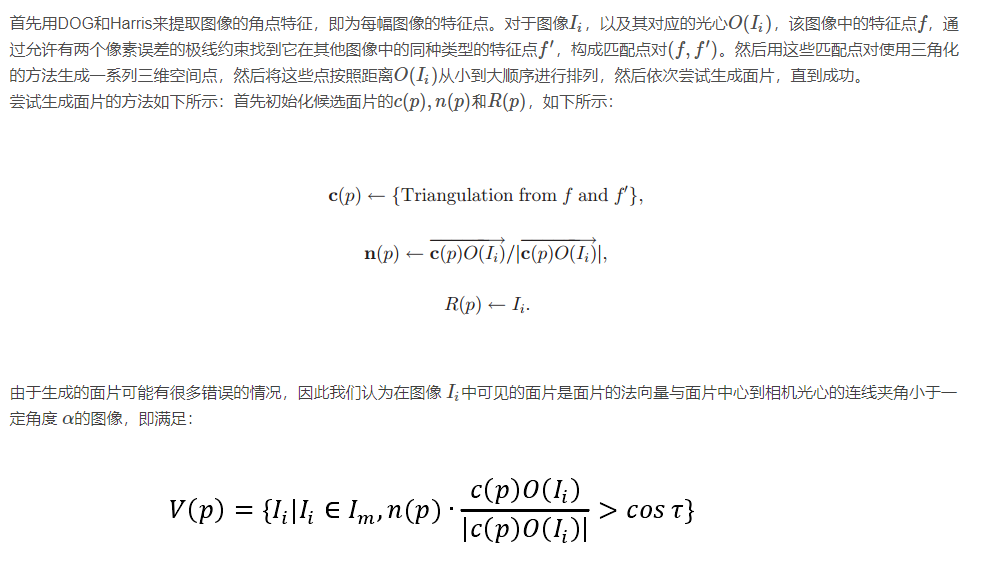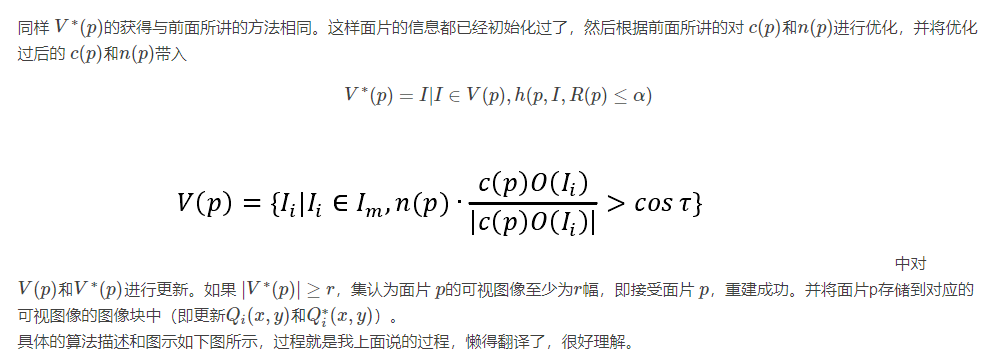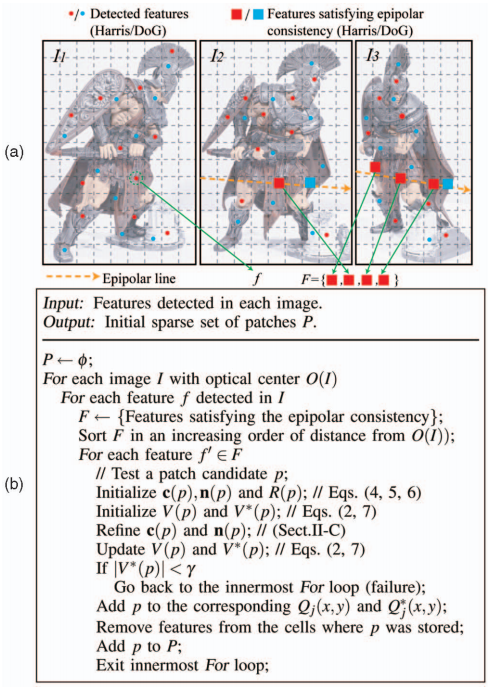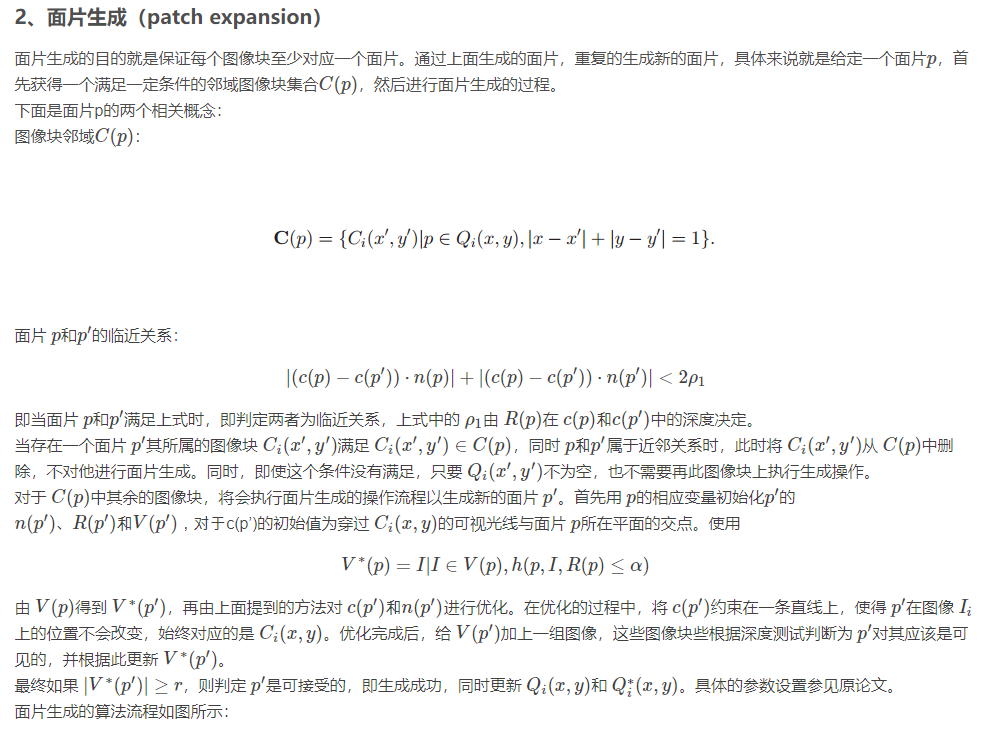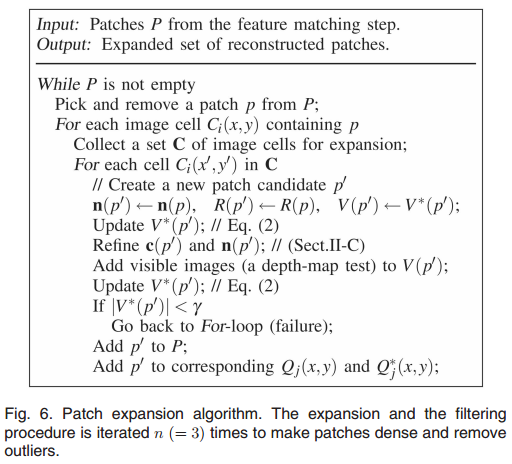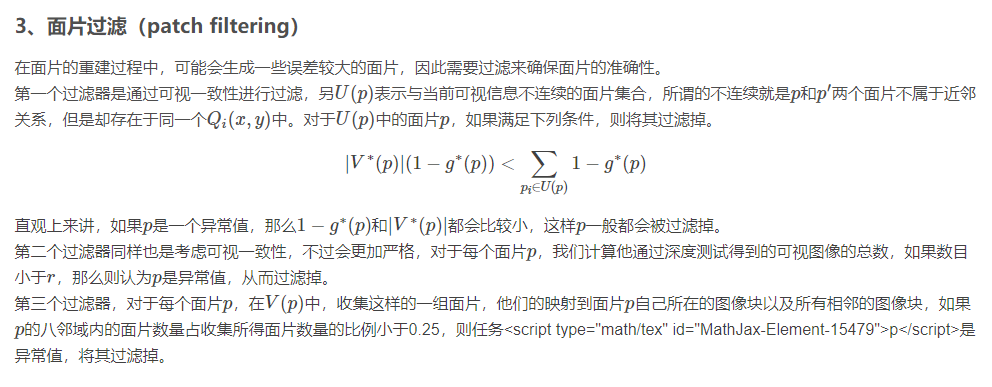CMVS算法概述：
CMVS目的 任务是将SFM的稀疏点聚类到不同的影像集（image cluster），每个影像集分别进行MVS算法。 这样做的好处是根据SFM稀疏点的信息对浩大的影像分块成多个小块，分别进行MVS，分治思想，减少MVS算法的资源峰值。
CMVS影像聚类的特点 1.删除多余影像（质量极差的影像） 2.保证cluster的size（保证每个cluster内影像数量小于某一size） 3.保证完整性和质量 完整性由每个特征点都会被cluster覆盖来保证 4.cluster之间有重叠
目标方程 为实现保证质量的目的，给出目标方程：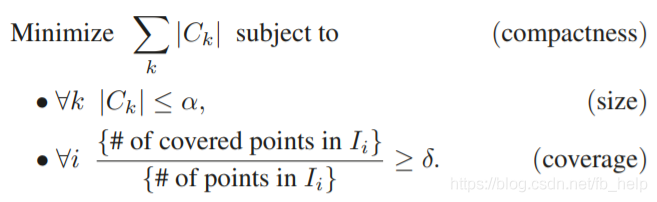目标方程中包含了：
1）最小化影像数量； 2）保证cluster大小； 3）保证收敛。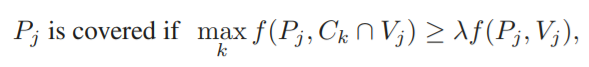1、点收敛； 2、影像收敛；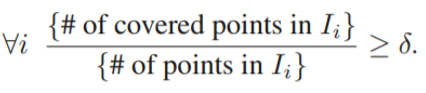衡量质量的标准（measurement）： 一个点P对应于一个影像集C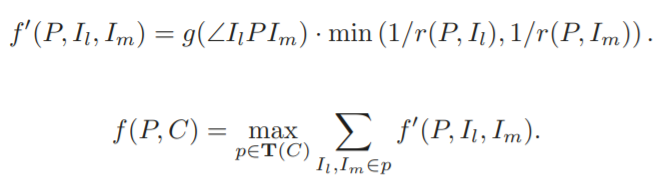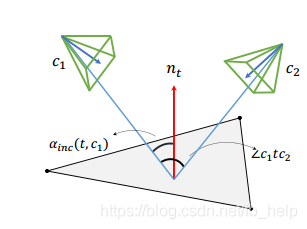方法步骤 1.SFM滤波—融合SFM点 2.除去质量不好的影像 3.使用normalized cut初始分割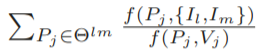边权：在这里插入图片描述 4.贪心的向初始分割中添加影像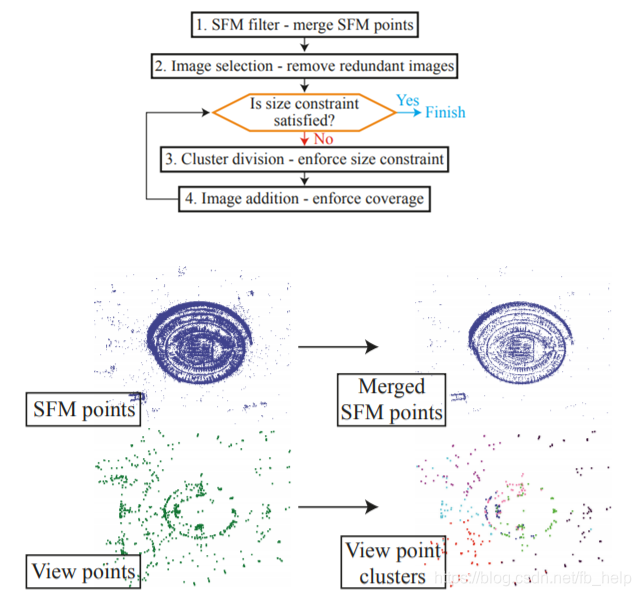展开全文• 下面详细介绍一下PMVS算法。一、基本概念介绍1、面片（patch）面片pp是一个近似的正切与重建物体表面的一个小矩形，他的一边平行于参考相机的x轴。对于一个面片pp，他的几何特征如下： 中心点：c(p)c(p)； 单位法...
通过前面的介绍，已经获得了相机的参数，我们可以利用这些参数使用基于面片的三维多视角立体视觉算法（PMVS）重建出稠密的点云。下面详细介绍一下PMVS算法。
一、基本概念介绍
1、面片（patch）
面片

p
$p$是一个近似的正切与重建物体表面的一个小矩形，他的一边平行于参考相机的x轴。对于一个面片p$p$，他的几何特征如下：  中心点：

c(p)
$c(p)$；  单位法向：

n(p)
$n(p)$，该向量指向相机的光心；  面片

p
$p$对应一个参考图像R(p)$R(p)$，在

R(p)
$R(p)$中

p
$p$是可见的。针对p$p$有扩展矩形，

p
$p$在R(p)$R(p)$中的投影是

μ×μ
$\mu \times \mu$大小的，在原论文中

μ=
$\mu =$5 or 7
2、灰度一致性函数（Photometric Discrepancy Function）
首先，我们另图像集合

V(p)
$V(p)$是所有在自身图像中可见面片

p
$p$的图像集合（显然这里有R(p)∈V(p)$R(p) \in V(p)$，将在后面介绍

V(p)
$V(p)$的获得已经

R(p)
$R(p)$的确定）那么，灰度一致性函数定义如下：

g(p)=1|V(p)−R(p)|∑I∈(V(p)−R(p))h(p,I,R(p)

g(p) = \frac {1}{|V(p) - R(p)|} \sum_{I \in (V(p) - R(p))}h(p,I,R(p)在这里，

V(p)−R(p)
$V(p) - R(p)$是指出去

R(p)
$R(p)$的

V(p)
$V(p)$的其他元素；

h(p,I1,I2)
$h(p,I_1,I_2)$是指

I和R(p)
$I和R(p)$的灰度一致性函数，计算过程如下：
1. 首先把面片

p
$p$划分为μ×μ$\mu \times \mu$的小格；
2. 通过双线性差值的方法，对

p
$p$在Ii$I_i$的图像上的投影进行差值，得到像素灰度

q(p,Ii)
$q(p,I_i)$；
3. 用1减去

q(p,I1)
$q(p,I_1)$和

q(p,I1)
$q(p,I_1)$的NCC(normalized cross correlation)值。
过程如下图所示：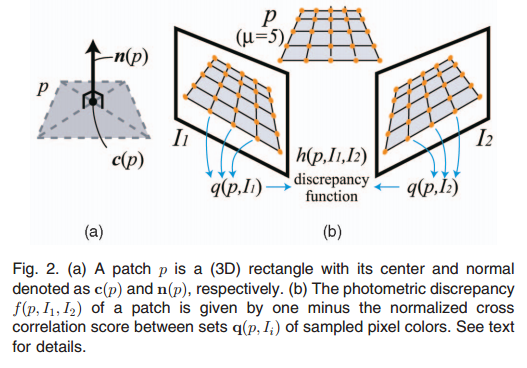由于

g(p)
$g(p)$对于图像中出现高光或者有障碍物的情况下的效果不好，因此在实际情况下，我们需要保证图像

I
$I$和图像R(p)$R(p)$的灰度一致性函数大于一定的值

α
$\alpha$（后面将会介绍如何选择这个阈值）。因此有：

V∗(p)=I|I∈V(p),h(p,I,R(p)≤α)

V^*(p) = {I|I \in V(p),h(p,I,R(p)\leq \alpha)}

g∗(p)=1|V∗(p)−R(p)|∑I∈(V∗(p)−R(p))h(p,I,R(p)

g^*(p) = \frac {1}{|V^*(p) - R(p)|} \sum_{I \in (V^*(p) - R(p))}h(p,I,R(p)

3、面片的优化
面片优化的目的就是恢复那些

g∗(p)
$g^*(p)$较小的面片，每个面片的重建过程分为以下两步：  4. 初始化面片的相关参数

c(p),n(p),V∗(p)和R(p)
$c(p),n(p),V^*(p)和R(p)$；  5. 优化

c(p)和n(p)
$c(p)和n(p)$  这里的第一步初始化的过程在后面用到，这里先讲以下

c(p)和n(p)
$c(p)和n(p)$的优化。几何参数

c(p)和n(p)
$c(p)和n(p)$的优化是通过最小化

g∗(p)
$g^*(p)$而得到的。为了简化计算，将

c(p)
$c(p)$约束在某一条光线上，这样

p
$p$在其对应的可视图集V∗(p)$V^*(p)$中某个图像的投影位置就不会变，因此降低了

p
$p$的自由度。n(p)$n(p)$是由欧拉角（yaw and pitch）决定的，可以用共轭梯度法求解这个优化问题。（这一段我没有懂这些方法）
4、图像模型
基于面片的物体表面的表示的优势是他的灵活性，然而却不容易找到面片的连续性。为了解决这个问题进行了如下的操作，把图像

Ii
$I_i$分成了许多

β1×β1
$\beta_1 \times \beta_1$像素的的小块

Ci(x,y)
$C_i(x,y)$，这里

x,y
$x,y$表示图像块的下标，

i
$i$表示这是第i$i$张图像的。给定一个面片

p
$p$和对应的V(p)$V(p)$，把p投影到

V(p)
$V(p)$的图像中，以得到面片p对应的图像块，每个图像块

Ci(x,y)
$C_i(x,y)$用一个集合

Qi(x,y)
$Q_i(x,y)$记录了所有投影到这个图像块的面片。同理，我们用

Q∗i(x,y)
$Q^*_i(x,y)$来表示用

V∗(p)
$V^*(p)$得到的结果。如下图所示：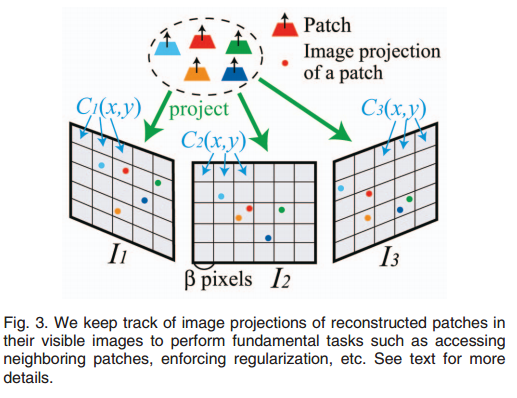二、面片的重构
基于面片的多视角三维立体视觉算法的目的就是保证在每个图像块

Ci(x,y)
$C_i(x,y)$上至少有一个面片的的投影。主要分为以下三步：  1. 初始化特征匹配（initial feature matching）；  2. 面片生成（patch expansion）；  3. 面片筛选（patch filtering）；  初始化特征匹配的目的就是生成一系列稀疏的面片，面片的生成和筛选都要执行n次使得面片足够稠密，同时去除不好的面片。下面依次进行介绍。
1、初始化特征匹配
首先用DOG和Harris来提取图像的角点特征，即为每幅图像的特征点。对于图像

Ii
$I_i$，以及其对应的光心

O(Ii)
$O(I_i)$，该图像中的特征点

f
$f$，通过允许有两个像素误差的极线约束找到它在其他图像中的同种类型的特征点f′$f'$，构成匹配点对

(f,f′)
$(f,f')$。然后用这些匹配点对使用三角化的方法生成一系列三维空间点，然后将这些点按照距离

O(Ii)
$O(I_i)$从小到大顺序进行排列，然后依次尝试生成面片，直到成功。  尝试生成面片的方法如下所示：首先初始化候选面片的

c(p),n(p)和R(p)
$c(p),n(p)和R(p)$，如下所示：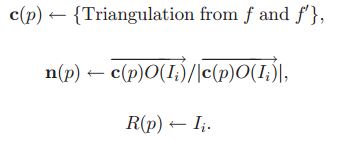由于生成的面片可能有很多错误的情况，因此我们认为在图像

Ii
$I_i$中可见的面片是面片的法向量与面片中心到相机光心的连线夹角小于一定角度

α
$\alpha$的图像，即满足：同样

V∗(p)
$V^*(p)$的获得与前面所讲的方法相同。这样面片的信息都已经初始化过了，然后根据前面所讲的对

c(p)和n(p)
$c(p)和n(p)$进行优化，并将优化过后的

c(p)和n(p)
$c(p)和n(p)$带入

V∗(p)=I|I∈V(p),h(p,I,R(p)≤α)

V^*(p) = {I|I \in V(p),h(p,I,R(p)\leq \alpha)}中对

V(p)和V∗(p)
$V(p)和V^*(p)$进行更新。如果

|V∗(p)|≥r
$|V^*(p)| \geq r$，集认为面片

p
$p$的可视图像至少为r$r$幅，即接受面片

p
$p$，重建成功。并将面片p存储到对应的可视图像的图像块中（即更新Qi(x,y)和Q∗i(x,y)$Q_i(x,y)和Q^*_i(x,y)$）。
具体的算法描述和图示如下图所示，过程就是我上面说的过程，懒得翻译了，很好理解。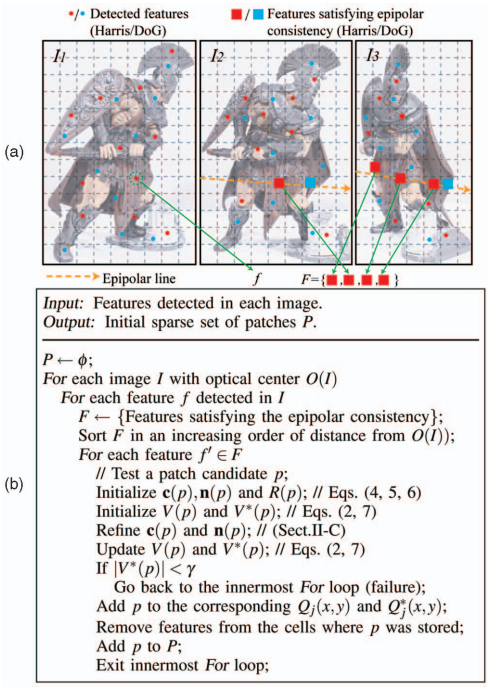2、面片生成（patch expansion）
面片生成的目的就是保证每个图像块至少对应一个面片。通过上面生成的面片，重复的生成新的面片，具体来说就是给定一个面片

p
$p$，首先获得一个满足一定条件的邻域图像块集合C(p)$C(p)$，然后进行面片生成的过程。  下面是面片p的两个相关概念：  图像块邻域

C(p)
$C(p)$：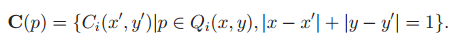面片

p
$p$和p′$p'$的临近关系：

|(c(p)−c(p′))⋅n(p)|+|(c(p)−c(p′))⋅n(p′)|<2ρ1

|(c(p) - c(p')) \cdot n(p)| + |(c(p) - c(p')) \cdot n(p')| < 2 \rho_1即当面片

p
$p$和p′$p'$满足上式时，即判定两者为临近关系，上式中的

ρ1
$\rho_1$由

R(p)
$R(p)$在

c(p)和c(p′)
$c(p)和c(p')$中的深度决定。
当存在一个面片

p′
$p'$其所属的图像块

Ci(x′,y′)
$C_i(x',y')$满足

Ci(x′,y′)∈C(p)
$C_i(x',y') \in C(p)$，同时

p和p′
$p和p'$属于近邻关系时，此时将

Ci(x′,y′)
$C_i(x',y')$从

C(p)
$C(p)$中删除，不对他进行面片生成。同时，即使这个条件没有满足，只要

Qi(x′,y′)
$Q_i(x',y')$不为空，也不需要再此图像块上执行生成操作。
对于

C(p)
$C(p)$中其余的图像块，将会执行面片生成的操作流程以生成新的面片

p′
$p'$。首先用

p
$p$的相应变量初始化p′$p'$的

n(p′)、R(p′)和V(p′)，对于
$n(p')、R(p')和V(p')，对于$c(p’)的初始值为穿过

Ci(x,y)
$C_i(x,y)$的可视光线与面片

p
$p$所在平面的交点。使用V∗(p)=I|I∈V(p),h(p,I,R(p)≤α)V^*(p) = {I|I \in V(p),h(p,I,R(p)\leq \alpha)}由

V(p)
$V(p)$得到

V∗(p′)
$V^*(p')$，再由上面提到的方法对

c(p′)和n(p′)
$c(p')和n(p')$进行优化。在优化的过程中，将

c(p′)
$c(p')$约束在一条直线上，使得

p′
$p'$在图像

Ii
$I_i$上的位置不会改变，始终对应的是

Ci(x,y)
$C_i(x,y)$。优化完成后，给

V(p′)
$V(p')$加上一组图像，这些图像块些根据深度测试判断为

p′
$p'$对其应该是可见的，并根据此更新

V∗(p′)
$V^*(p')$。
最终如果

|V∗(p′)|≥r
$|V^*(p')| \geq r$，则判定

p′
$p'$是可接受的，即生成成功，同时更新

Qi(x,y)
$Q_i(x,y)$和

Q∗i(x,y)
$Q_i^*(x,y)$。具体的参数设置参见原论文。
面片生成的算法流程如图所示：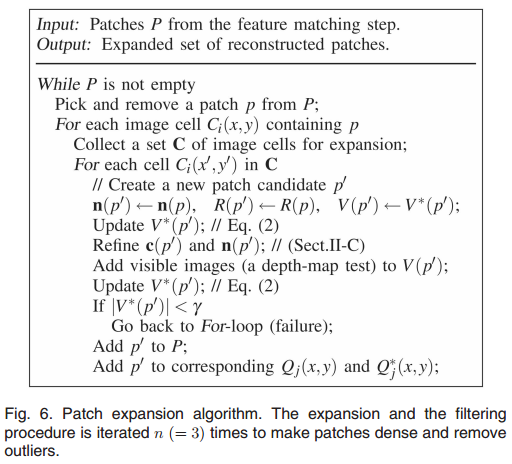3、面片过滤（patch filtering）
在面片的重建过程中，可能会生成一些误差较大的面片，因此需要过滤来确保面片的准确性。  第一个过滤器是通过可视一致性进行过滤，另

U(p)
$U(p)$表示与当前可视信息不连续的面片集合，所谓的不连续就是

p
$p$和p′$p'$两个面片不属于近邻关系，但是却存在于同一个

Qi(x,y)
$Q_i(x,y)$中。对于

U(p)
$U(p)$中的面片

p
$p$，如果满足下列条件，则将其过滤掉。|V∗(p)|(1−g∗(p))<∑pi∈U(p)1−g∗(p)|V^*(p)|(1 - g^*(p)) < \sum_{p_i \in U(p)}1 - g^*(p)直观上来讲，如果

p
$p$是一个异常值，那么1−g∗(p)$1 - g^*(p)$和

|V∗(p)|
$|V^*(p)|$都会比较小，这样

p
$p$一般都会被过滤掉。
第二个过滤器同样也是考虑可视一致性，不过会更加严格，对于每个面片p$p$，我们计算他通过深度测试得到的可视图像的总数，如果数目小于

r
$r$，那么则认为p$p$是异常值，从而过滤掉。  第三个过滤器，对于每个面片

p
$p$，在V(p)$V(p)$中，收集这样的一组面片，他们的映射到面片

p
$p$自己所在的图像块以及所有相邻的图像块，如果p$p$的八邻域内的面片数量占收集所得面片数量的比例小于0.25，则任务

p
<script type="math/tex" id="MathJax-Element-15479">p</script>是异常值，将其过滤掉。
展开全文三维重建
• ## PMVS算法原理总结

千次阅读 2020-10-14 09:48:07
这里写自定义目录标题欢迎使用Markdown编辑器新的改变功能快捷键合理的创建标题，有助于目录的生成如何改变文本的样式插入链接与图片如何插入一段漂亮的代码片生成一个适合你的列表创建一个表格设定内容居中、居左、...
一、理论基础 表面切片模型： 在计算机视觉领域，我们可以用场景的局部切平面来描述场景的表面，这些局部切平面被称为表面切片。 我们可以用一个矩形来描述表面切片，每个表面切片都附带有中心坐标c§以及一个单位法向量n§来表征表面切片的位置和朝向。 对于每个表面切片p都赋予一个参考图像R§，一个可见图像集合V§以及真正可见图像集合T§，参考图像为相机光轴与表面切片法向量夹角最小的图像，并且表面切片的法向量指向该参考图像，可见图像集合V§表示那些理论上看到表面切片p的图像集合，但实际上由于遮挡等原因可能看不到表面切片，所以T§的作用就是记录真正可以看到表面切片p的图像集合。 另外，令参考图像的x轴与表面切片p的x轴近似平行。表面切片的几何结构如图所示：二、PMVS算法描述 PMVS算法中将每张图像分成许多𝛽1× 𝛽1像素大小的图像块𝐶(𝑥, 𝑦)，每个图像块用一个集合𝑄(𝑥, 𝑦)来记录所有投影到这个图像块的表面切片。基于表面切片的PMVS算法目的就是保证每个图像块𝐶(𝑥, 𝑦)上都至少有一个表面切片的投影。 其过程主要分为三个步骤： （1）初始化特征匹配 （2）表面切片的生成 （3）表面切片的过滤 通过特征匹配并三角化后既可以初始化得到稀疏表面切片，之后生成更多的表面切片并对错误的表面切片进行过滤，这两个过程迭代多次来生成更多的表面切片并对错误的表面切片进行过滤。 PMVS 算法中使用 DoG 算子和 Harris 角点提取算子来提取图像的特征，之后对特征点进行匹配，通过三角化的方式可以根据匹配点对生成稀疏空间点云，然后将这些点按照点到对应相机光心的距离从小到大排序，依次尝试生成表面切片直到成功为止，初始化后得到初始表面切片。 之后根据这些表面切片重复生成新的表面切片，具体来说就是对每一个表面切片，首先定义其满足一定条件的邻域图像集合，在此集合中尝试生成新的表面切片。 最后是对表面切片进行过滤来剔除错误的表面切片PMVS 算法中使用了三个过滤器，一个是通过 可见一致性标准来过滤，剔除掉不满足可视一致性标准的表面切片；另一个过滤器也考虑了可见一致性，对于每个表面切片计算其通过深度测试的可见图像总数，剔除那些总数没有超过一定阈值的表面切片；最后的过滤器过滤掉那些孤立的表面切片。
展开全文• 下面详细介绍一下PMVS算法。 一、基本概念介绍 1、面片（patch） 面片p是一个近似的正切与重建物体表面的一个小矩形，他的一边平行于参考相机的x轴。对于一个面片p，他的几何特征如下：中心点：c(p)；单位法向：n...

通过前面的介绍，已经获得了相机的参数，我们可以利用这些参数使用基于面片的三维多视角立体视觉算法（PMVS）重建出稠密的点云。下面详细介绍一下PMVS算法。
一、基本概念介绍
1、面片（patch）
面片
2、灰度一致性函数（Photometric Discrepancy Function）
首先，我们定义图像集合

g(p)=1\|V(p)\R(p)|∑I∈(V(p)\R(p))h(p,I,R(p)

在这里，V∗(p)={I|I∈V(p),h(p,I,R(p)≤α)}

g∗(p)=1\|V∗(p)\R(p)|∑I∈(V∗(p)\R(p))h(p,I,R(p)

3、面片的优化
面片优化的目的就是恢复那些
4、图像模型
基于面片的物体表面的表示的优势是他的灵活性，然而却不容易找到面片的连续性。为了解决这个问题进行了如下的操作，把图像二、面片的重构
基于面片的多视角三维立体视觉算法的目的就是保证在每个图像块
1、初始化特征匹配
首先用DOG和Harris来提取图像的角点特征，即为每幅图像的特征点。对于图像V∗(p)=I|I∈V(p),h(p,I,R(p)≤α)2、面片生成（patch expansion）
面片生成的目的就是保证每个图像块至少对应一个面片。通过上面生成的面片，重复的生成新的面片，具体来说就是给定一个面片|(c(p)−c(p′))⋅n(p)|+|(c(p)−c(p′))⋅n(p′)|<2ρ1

V∗(p)=I|I∈V(p),h(p,I,R(p)≤α)3、面片过滤（patch filtering）
在面片的重建过程中，可能会生成一些误差较大的面片，因此需要过滤来确保面片的准确性。 第一个过滤器是通过可视一致性进行过滤，另

|V∗(p)|(1−g∗(p))<∑pi∈U(p)1−g∗(p)

权重n(p)是C(p)和线距离的高斯函数标准偏差ρ1定义在3.2.1中，归一化总和为1.8
4.2 网格细化
所有顶点的是三维坐标被优化和关于每个顶点光度一致性和几何平滑能量函数总和
1）一个表面的深度和方向被估计，它的每对可视化图像集相对每个顶点通过用面片优化过程
2）评估深度和方向信息被联合计算能量函数
V(vi)表示一组图像集，其中vi是可视化的图像，从一个标准深度图测试集评估的当前网格模型
E'p(vi)=ζ3∑p∈P(vi)1-exp(-(d'(vi,p)/τ/4)^2)
d'(vi,p)=n(p)*(c(p)-vi)
其中d‘(vi,p)是面片p和顶点vi沿着单位面片的符号距离，τ是网格平均加权长度，ζ3是线性组合权重。

（以上是我的修改和补充的部分，如有错误请指正，我会继续修改直到最好。。。。。。）

转载于:https://www.cnblogs.com/yuan328/p/6430023.html
展开全文• 针对PMVS算法在多视倾斜影像密集匹配中的不足,结合城市三维建模的物方特点,将高程约束条件、聚类分析方法和候选影像排序策略引入其中,并用格网扩散代替原算法中的六方向扩散,从而形成了一种适合大倾斜影像的PMVS改进...
• 基于PMVS算法的三维重建实现，使用c++编写，简练直观，可得到较好的3D图像
• ## PMVS学习中学习c++

千次阅读 2014-02-16 20:51:39
最近忙于PMVS算法的优化，在这个过程中把这个写下来。仿照已有PMVS源程序，给出自己的一个实现过程。 1、fstream的使用 2、c++中的初始化 3、new创建对象与不用new的区别   我们学习这个c++的时候，一般用...
• 下面这个方法适用于那些只想对稠密重建算法CMVS-PMVS进行研究，而不想在前期bundler、cygwin的配置操作上浪费时间的学者 ======================================================================================...CMVS Bundler 三维重建
• ## PMVS详细讲解

热门讨论 2014-01-13 10:52:39
pmvs是基于面片的三维立体重建算法，有具体的源代码。
• 介绍目前精度和完整性较高的多视角三维重建算法，（patch-based multi-view stere0,PMVS
• 基于面片的多视图稠密重建方法（PMVS）能够自动忽略外部点和障碍点，相比较于其他三维稠密重建算法算法更准确，简单，高效.但是在纹理稀疏的区域会出现孔洞残缺等问题，且现有的匹配候选点选取策略会使得局部细节...
• PMVS的中文讲解ppt（原文Accurate, Dense, and Robust Multiview Stereopsis）讲解很详细，易懂
• 最近花了两三个星期左右的时间看PMVS的东西，第一遍看原始论文感觉完全串不起来流程，就是翻译了一遍英文。然后被老师训了两个星期没成果（其中一个星期十一假期在玩>.<），下来后又开始啃论文，理了一遍流程...
• 运用SIFT算法进行特征提取，通过匹配图像之间的特征点，利用SfM算法对匹配结果进行光束法平差，得到相机的姿态和稀疏点云，最终采用多视密集匹配方法PMVS实现场景目标的密集重建。 实现软件为VisualSFM+PMVS。 ...sift
•cygwin bundler
• ## CMVS-PMVS 知识库链接

千次阅读 2017-08-27 19:53:05
三维重建（四）PMVS算法 the patch-based MVS algorithm 特征点检测——BRISK算法 特征点匹配——FREAK算法介绍 特征点匹配——使用基础矩阵、单应性矩阵的RANSAC算法去除误匹配点对 特征点匹配应用——图像...
• 首先构建一个空的项目pmvs，控制台应用程序，然后加pmvs2下的base下的三个文件夹拷贝到项目下，然后对应头文件及源文件添加到项目中。改写新建项目中的pmvs.cpp,将pmvs2中main里面的pmvs2.cc里面的部分内容添加到...
• Windows下使用VisualSFM + CMVS/PMVS +MeshLab进行三维重建 参考文档： VisualSFM和Meshlab实现三维重建过程：http://planckscale.info/tag/visualsfm/（十分感谢） 数据集： 三维重建数据集：...三维重建 VisualSFM MeshLab
• PMVS NCC(Normalized Cross Correlation)归一化互相关 一：基本原理 NCC是一种基于统计学计算两组样本数据相关性的算法，其取值范围为[-1, 1]之间，而对图像来说，每个像素点都可以看出是RGB数值，这样整幅图像就...
• 基于面片的多视角立体(PMVS)算法只需要输入一组图片及摄像机参数便可生成稠密的带有颜色的三维点云,它无需任何的初始信息。但该算法仍有一些不足,一方面重建出的表面不够光滑和连续,特别是在大场景重建时经常碰到的...计算机技术与应用
• 参考博文： 1.https://www.jianshu.com/p/ef3ef6fdf354 2.https://blog.csdn.net/u013358387/article/details/71576660 3.https://blog.csdn.net/zouyu1746430162/article/details/78639469 ...
• pmvs算法组织清晰明了，原创作者的论文《Accurate, Dense, and Robust Multi-View Stereopsis》对该算法的原理介绍的十分清楚，该算法分为三个阶段：特征匹配，扩散，过滤，这三个阶段涉及的有patch模型，image模型...
• 研究三维重建相关的课题，记录一些内容在这儿 通用的体验流程： visualSFM + pmvs + meshlab visualSFM通过找到一些列的图像的特征，重构出3D模型的稀疏点云 ...pmvs算法组织清晰明了，原创作者的论文...
• pmvs2----------") pRecons = subprocess.Popen( [os.path.join(PMVS_BIN, "pmvs2"), reconstruction_dir+"/PMVS/", "pmvs_options.txt"] ) # 注：不要修改pmvs_options.txt文件名 pRecons.wait() #*注：执行后会在...人工智能 python 编程语言 计算机视觉 java
• 接着，通过实时优化调整原始ＰＭＶＳ算法密集匹配中的面片法向，并采用分层重建的策略，提高了重建密集点云的法向一致性与时间效率；最后，通过一定的网格简化策略，获得Ｈ维城市场景的Ｐｏｉｓｓｏｎ网格表面模型。...
• 三维计算机视觉在计算机视觉是偏基础的方向，随着2010年阿凡达在全球热映以来，三维计算机视觉的应用从传统工业领域逐渐走向生活、娱乐、服务等，比如AR/VR，SLAM，...图 1基于图像的三维重建算法SfM（Structure Fr...
• 使用openMVG+PMVS实现视觉三维重建 一、什么是视觉三维重建？ 我们知道，照相机的原理是将一个三维场景投影到二维平面。所谓视觉三维重建，顾名思义就是从已有的二维图像中恢复原始三维场景。 三维重建的原理大致......